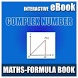# MATHS-COMPLEX NUMBER-FORMULA EBOOKFree App

Rated 0.00/5 (0) —  Free Android application by Learn with Quiz

Complex Number Interactive e-Book for IIT-JEE & other Competitive Exams.

Board: CBSE, GSEB, ICSE, ISC, International Board, Cambridge
Class: 11th - 12th Students, Engineering Students and Medical Students.

Maths (AIEEE, IIT-JEE Main) Complex Number Interactive e-Book Education application
Topic:
Basic Concepts of Complex Number
Real and Imaginary Parts of a Complex Number
Algebraic Operations with Complex Numbers
Properties of algebraic operations on complex numbers
Equality of Two Complex Numbers
Conjugate of a complex number
Modulus of a Complex Number
Properties of Modulus
Argument of a Complex Number
Square Root of a Complex Number
Various Representations of a Complex Number
Logarithm of a Complex Number
Use of Complex numbers in Co-ordinate Geometry
Rotation Theorem
Triangle Inequalities
Standard loci in the Argand Plane
De' Moivre's Theorem
Summation of series ‘C + is’ Method
Roots of a Complex Number
Points at a Glance

Free
downl.
Android
5.0+
For everyone
Android app

What's Changed
Update Graphics and Content

Oh snap! No comments are available for MATHS-COMPLEX NUMBER-FORMULA EBOOK at the moment. Be the first to leave one!

RATING
0.05
0 users

5

4

3

2

1